# DAV Class 4 Maths Chapter 9 Worksheet 1 Solutions

The DAV Class 4 Maths Solutions and DAV Class 4 Maths Chapter 9 Worksheet 1 Solutions of Fractions offer comprehensive answers to textbook questions.

## DAV Class 4 Maths Ch 9 WS 1 Solutions

Question 1.
Represent the equivalent fractions $$\frac{1}{2}, \frac{2}{4}, \frac{4}{8}$$ in the given figures.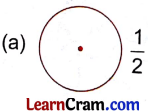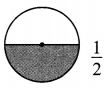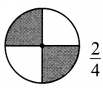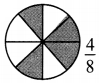Question 2.
Represent the equivalent fractions $$\frac{1}{3}, \frac{2}{6}, \frac{4}{12}$$ in the given figures.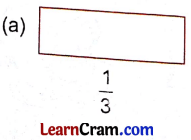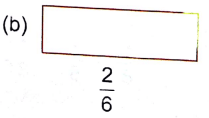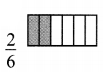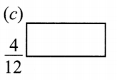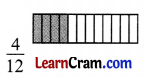### DAV Class 4 Maths Chapter 9 Worksheet 1 Notes

• ‘Fraction’ means a ‘part’ or a ‘fragment’
• One half – 1 part out of 2 equal parts is taken. So when an object is divided into two equal parts, then each part is called one half of the whole. It is expressed as $$\frac{1}{2}$$.
• One-third -1 part out of 3 equal parts is taken. We express one-third as $$\frac{1}{3}$$.
• Two-third -2 parts out of 3 equal parts are taken. It is expressed as $$\frac{2}{3}$$.
• One-fourth – 1 part out of 4 equal parts is taken. It is expressed as $$\frac{1}{4}$$.
• We read them as ‘One by two’, ‘one by three’ two by three’, and one by four.

Question 1.
Write fraction for the shaded part of each group.$$\frac{5}{12}$$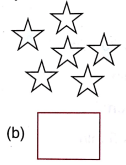$$\frac{2}{6}$$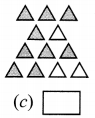$$\frac{9}{12}$$

Question 2.
Shade the figure according to the give fraction.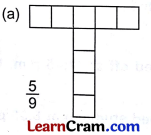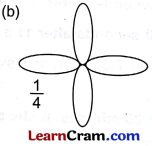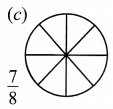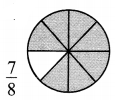Question 3.
Complete the table:

 Numerator Denominator Fraction (a) 5 8 _____ (b) 3 17 _____ (c) 6 ____ $$\frac{6}{10}$$ (d) _____ 4 $$\frac{1}{4}$$

(a) $$\frac{5}{8}$$
(b) $$\frac{3}{17}$$
(c) 10
(d) 1

Question 4.
Compare using ‘<‘, ‘>’ ‘=’
(a) $$\frac{4}{3}$$ ______ $$\frac{7}{3}$$
<

(b) $$\frac{1}{8}$$ ______ $$\frac{1}{8}$$
=

(c) $$\frac{13}{17}$$ ______ $$\frac{5}{17}$$
>

(d) $$\frac{3}{10}$$ ______ $$\frac{3}{10}$$
=Question 5.
Solve the following:
(a) $$\frac{4}{9}+\frac{1}{9}$$
$$\frac{4}{9}+\frac{1}{9}$$
= $$\frac{4+1}{9}$$
= $$\frac{5}{9}$$

(b) $$\frac{2}{11}+\frac{5}{11}+\frac{3}{11}$$
$$\frac{2}{11}+\frac{5}{11}+\frac{3}{11}$$
= $$\frac{2+5+3}{11}$$
= $$\frac{\mathbf{1 0}}{\mathbf{1 1}}$$

(c) $$\frac{7}{8}-\frac{2}{8}$$
$$\frac{7}{8}-\frac{2}{8}$$
= $$\frac{7-2}{8}$$
= $$\frac{5}{8}$$

(d) $$\frac{13}{17}-\frac{9}{17}$$
$$\frac{13}{17}-\frac{9}{17}$$
= $$\frac{13-9}{17}$$
= $$\frac{4}{17}$$

(e) $$\frac{4}{10}+\frac{3}{10}+\frac{1}{10}$$
$$\frac{4}{10}+\frac{3}{10}+\frac{1}{10}$$
= $$\frac{4+3+1}{10}$$
=\frac{8}{10}[/latex]

(f) $$\frac{15}{13}-\frac{3}{13}$$
$$\frac{15}{13}-\frac{3}{13}$$
= $$\frac{15-3}{13}$$
= $$\frac{12}{13}$$
Example: $$\left(\frac{1}{3}, \frac{2}{6}, \frac{4}{12}\right)$$
Thus, $$\frac{1}{3}=\frac{2}{6}=\frac{4}{12}$$ are equivalent fractions.